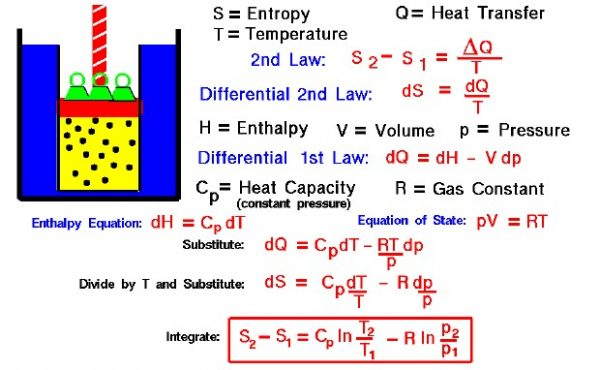# Entropy of a GasThermodynamics is a branch of physics that deals with the energy and work of a system. As aerodynamicists, we are most interested in thermodynamics in the study of propulsion systems. The first law of thermodynamics indicates that the total energy of a system is conserved. This includes the potential and kinetic energy, the work done by the system, and the transfer of heat through the system. The second law of thermodynamics indicates that, while many physical processes that satisfy the first law are possible, the only processes that occur in nature are those for which the entropy (S) of the system either remains constant or increases. Entropy, like temperature (T) and pressure (p), is a state variable of the system. Entropy is defined to be the heat transfer (delta Q) into the system divided by the temperature. For a process going from state 1 to state 2:

### S2 – S1 = delta Q / T

During a thermodynamic process, the temperature of an object changes as heat is applied or extracted. So a more correct definition of the entropy is the differential form that accounts for this variation.

### dS = dQ / T

The change in entropy is then the inverse of the temperature integrated over the change in heat transfer. For gases, there are two possible ways to evaluate the change in entropy. The equations can be formulated in terms of the internal energy (E) and the work (W) for a gas. Or, it may be formulated in terms of the enthalpy (H) of the gas. We shall present here the enthalpy formulation because we will use the entropy relations to determine how the temperature changes for a change in pressure inside a cylinder of the Wright 1903 engine.

Let us begin by deriving a differential form of the first law of thermodynamics. The first law is given by:

### E2 – E1 = Q – W

The differential form would be:

### dE = dQ – dW

For a gas, the work is given by

Substituting:

### dE = dQ – p dV

From the definition of enthalpy:

### dE = dH – p dV – V dp

Substitute into the first law equation:

### dH – V dp = dQ

Using the equation of state, we can modify the second term of this equation in terms of the gas constant, the pressure, and the temperature.

### dH – (R * T) dp / p = dQ

The enthalpy of a gas is equal to the specific heat of constant pressure times the change in temperature; in differential form:

### Cp dT – (R * T) dp / p = dQ

Divide by the temperature and substitute dS = dQ / T :

### Cp dT / T – R dp / p = dS

This is a differential equations which we can easily integrate.

### S2 – S1 = Cp * ln ( T2 / T1) – R * ln ( p2 / p1)

The “ln” is the natural logarithm function which you find on your calculator and which is given in tables in math books.

What good is all of this?

There are several processes which take place within an engine for which there is no transfer of heat into the system. A simple compression is one of these adiabatic processes. If there is no heat transfer, the change of entropy is zero and we have a process that is reversible. The equation derived above relates the change in temperature to the change in pressure. For a given compression ratio, we can determine how hot the gas becomes.

Provide feedback